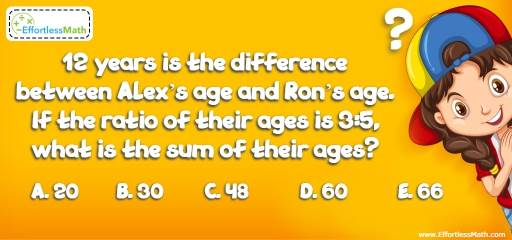# Algebra Puzzle – Challenge 33

Who can solve this great math challenge? It is for those who enjoy challenging their brains!## Challenge:

12 years is the difference between Alex’s age and Ron’s age. If the ratio of their ages is 3:5, what is the sum of their ages?

A- 20

B- 30

C- 48

D- 60

E- 66

### The Absolute Best Book to challenge your Smart Student!

Let A be Alex’s age and R be Ron’s age. The ratio of their ages is 3 to 5 and the difference between their ages is 12 years. Therefore, $$R = A + 12$$ and $$A = \frac{3}{5} R$$
Replace A in the first equation with its value in the second equation.
$$R = A + 12 → R = \frac{3}{5} R + 12 → \frac{2}{5} R = 12 → R = 30$$ and $$A = 18$$
The sum of their ages is 48.

### What people say about "Algebra Puzzle – Challenge 33 - Effortless Math: We Help Students Learn to LOVE Mathematics"?

No one replied yet.

X
30% OFF

Limited time only!

Save Over 30%

SAVE $5 It was$16.99 now it is \$11.99